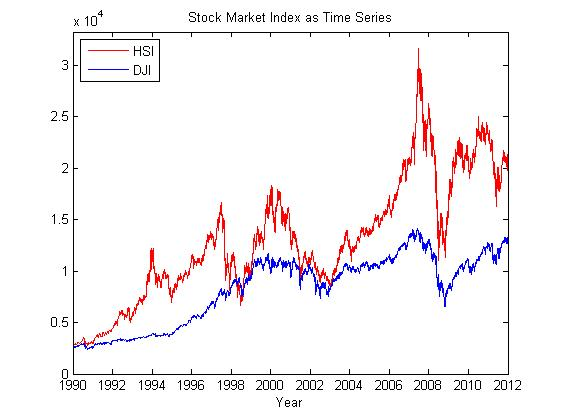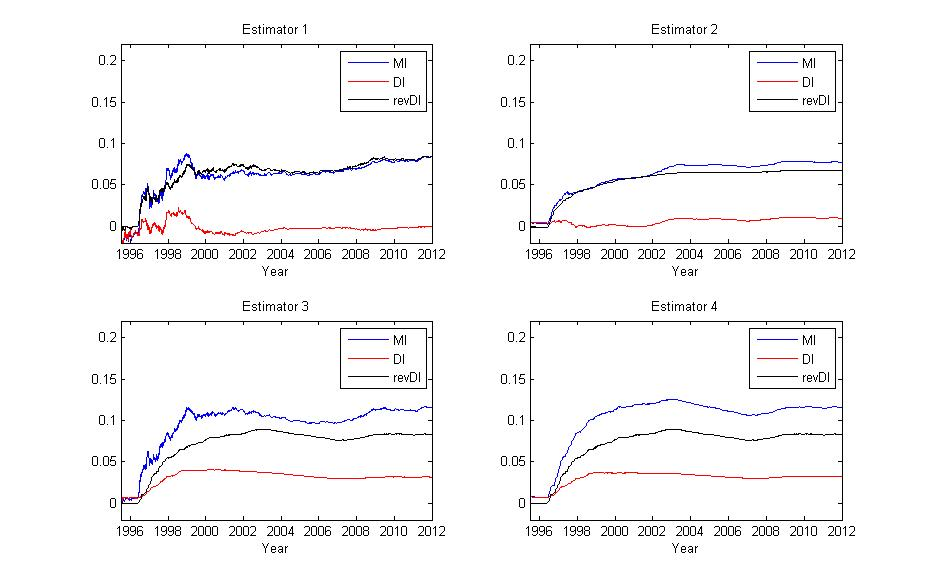Universal Estimation of Directed Information

DI Code Release:  This matlab package includes two main functions 'compute_DI_MI.m', 'ctwprob.m', several auxiliary functions, four examples programs, and a 'userguide.pdf' document. The four example programs correspond to four examples in the Section V of paper "Universal estimation of directed information", IEEE Transactions on Information Theory 59, no. 10 (2013): 6220-6242.

Installation and Execution

1. Call the function 'compute_DI_MI' with the following format:

[MI, DI, rev_DI]=compute_DI_MI(X,Y,Nx,D,alg,shift_ratio,prob,flag)

1. 'X' and 'Y' are input sequences with the same length;
2. 'Nx' is the size of the alphabet of process X, with the assumption that processes X and Y have the same size of alphabets;
3. 'D' is the maximum depth of the context tree used in basic CTW method;
4. 'alg' is a string that indicates which directed information estimator in paper 'Universal Estimation of Directed Information' is used, namely 'E1', 'E2', 'E3', 'E4'.
5. 'prob' is a struct containing probability assignments generated by function 'ctwprob' in case users want to avoid running CTW many times on the same data sequences;
6. 'flag' indicates whether 'compute_DI_MI' calculates the CTW probability assignment. If 'flag' = 0, then the input parameter 'prob' is taken as invalid, function 'compute_DI_MI' calculates CTW probability assignment itself; else the input parameter 'prob' is valid and 'compute_DI_MI' doesn't compute CTW probability assignment but directly take it from the input parameter 'prob';
7. 'MI' is a vector of estimated mutual information I(X^n;Y^n) for different n;
8. 'DI' is a vector of estimated directed information I(X^n-->Y^n) for different n;
9. 'rev_DI' is a vector of estimated reverse directed information I(Y^{n-1}-->X^n) for different n;
10. 'shift_ratio' determines what proportion of the estimated directed information will be discarded when generating the outputs. For example, if 'shift_ratio' = 0.3, and the length of input process X is 1000, then output 'MI' will have length of (1-0.3)*1000 = 700, and it only gives the estimated I(X^n; Y^n) for n not smaller than 300, so do 'DI' and 'rev_DI'.

2. Call the function 'ctwprob' with the following format:

[prob] = ctwprob(X,Y,Nx,D)

where 'X','Y','Nx','D' are of the same meanings as shown above.